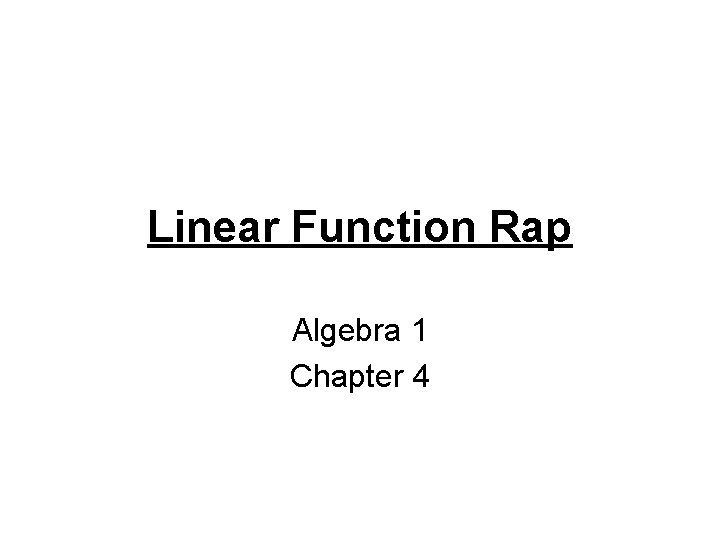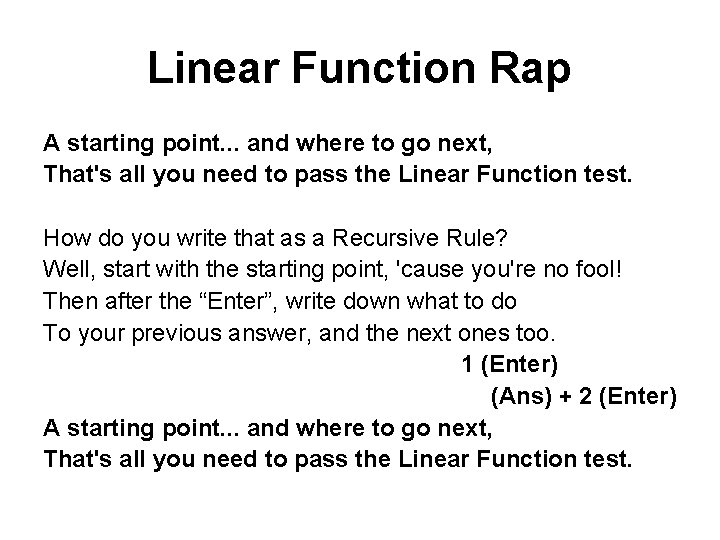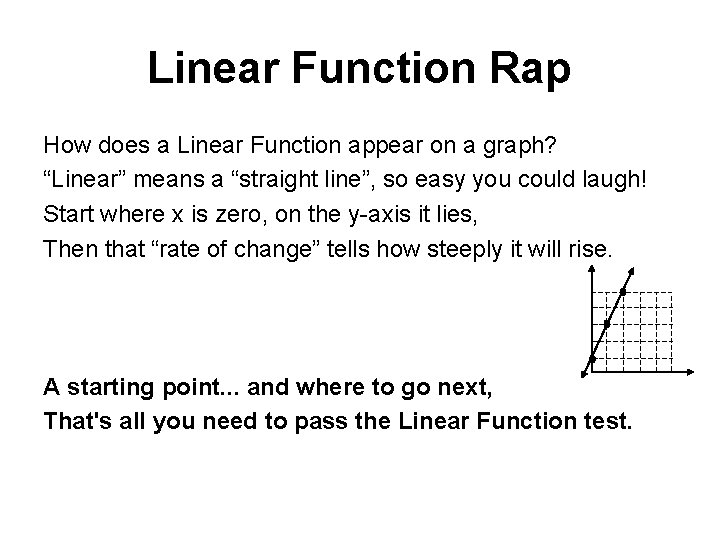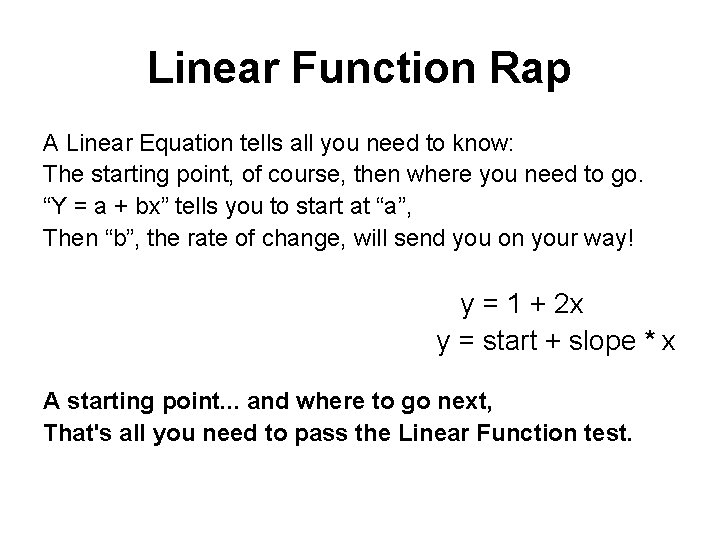# Linear Function Rap Algebra 1 Chapter 4 Linear

• Slides: 6Linear Function Rap Algebra 1 Chapter 4Linear Function Rap A starting point. . . and where to go next, That's all you need to pass the Linear Function test. How do you write that as a Recursive Rule? Well, start with the starting point, 'cause you're no fool! Then after the “Enter”, write down what to do To your previous answer, and the next ones too. 1 (Enter) (Ans) + 2 (Enter) A starting point. . . and where to go next, That's all you need to pass the Linear Function test.Linear Function Rap What does Linear look like when seen on a table? It starts where x is zero and that's no fable. Then it goes up steadily, a constant rate of change. Rise over run—that's not so strange! x 0 1 2 y 1 3 5 3 7 A starting point. . . and where to go next, That's all you need to pass the Linear Function test.Linear Function Rap How does a Linear Function appear on a graph? “Linear” means a “straight line”, so easy you could laugh! Start where x is zero, on the y-axis it lies, Then that “rate of change” tells how steeply it will rise. A starting point. . . and where to go next, That's all you need to pass the Linear Function test.Linear Function Rap A Linear Equation tells all you need to know: The starting point, of course, then where you need to go. “Y = a + bx” tells you to start at “a”, Then “b”, the rate of change, will send you on your way! y = 1 + 2 x y = start + slope * x A starting point. . . and where to go next, That's all you need to pass the Linear Function test.Linear Function Rap A starting point. . . and where to go next, That's all you need to pass the Linear Function test. 1 (Enter) (Ans) + 2 (Enter) y = 1 + 2 x y = start + slope * x x 0 1 2 y 1 3 5 3 7 A starting point. . . and where to go next, That's all you need to pass the Linear Function test.